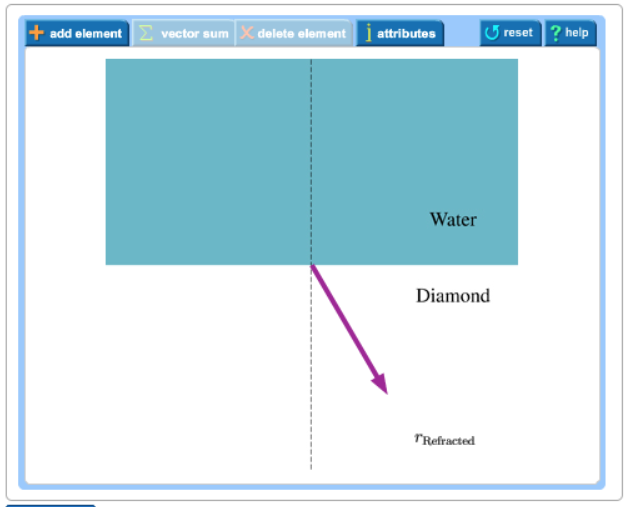# Problem: Learning goal:To practice Tactics Box 18.1 Analyzing refraction. If a ray refracts between medium 1 and medium 2, having indices of refraction n1 and n2, the ray angles θ1 and θ2 in the two media are related by Snell's law'n1 sin  θ1 = n2 sin θ2. This law becomes a central idea in a procedure for analyzing refraction problems, as explained in the following Tactics Box. Below is a ray diagram showing the refracted ray as it travels across the diamond. The dashed line represents the normal to the surface between the water and the diamond. Complete the diagram by adding the incident ray. Even though you don't know the exact direction of the incident ray yet, you can estimate it by applying step 3 in the Tactics Box above. Use your estimate to complete the ray diagram. The location and orientation of the vector will be graded. The length of the vector will not be graded.

###### FREE Expert Solution

From the relation, n1 sin  θ1 = n2 sin θ2

the incident ray will bend toward the normal when it travels from a less optically dense medium to an optically denser medium.###### Problem Details

Learning goal:

To practice Tactics Box 18.1 Analyzing refraction. If a ray refracts between medium 1 and medium 2, having indices of refraction n1 and n2, the ray angles θ1 and θ2 in the two media are related by Snell's law'

n1 sin  θ1 = n2 sin θ2

This law becomes a central idea in a procedure for analyzing refraction problems, as explained in the following Tactics Box.

Below is a ray diagram showing the refracted ray as it travels across the diamond. The dashed line represents the normal to the surface between the water and the diamond. Complete the diagram by adding the incident ray. Even though you don't know the exact direction of the incident ray yet, you can estimate it by applying step 3 in the Tactics Box above. Use your estimate to complete the ray diagram. The location and orientation of the vector will be graded. The length of the vector will not be graded.